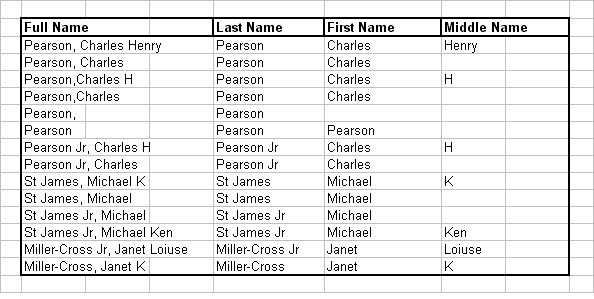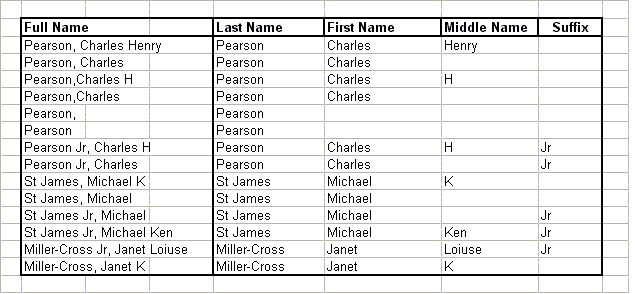Extracting First And Last Names

 A frequent task many people encounter when working with lists of data in Excel is splitting full names into the first-name and last name components. For example, a user may have full names like "Pearson, Charles H" in column A, and needs to put the last name in column B, the first name in column C, and the middle initial in column D.   This page describes some worksheet formulas and VBA procedures you can use to accomplish this.  For a related procedure for working with telephone numbers, see Parsing Telephone Numbers.  All of the functions and procedures on this page assume that your data is formatted as "LastName, FirstName MiddleName".  For example, "Pearson, Charles H".  Suffixes like "Jr" are permitted in the LastName, as are two word last names (e.g., St James) and hyphenated last names (e.g., Smith-Cross).  For example, all the the following names are valid.   Pearson Pearson, Charles Pearson, Charles H Pearson, Charles Henry Pearson Jr, Charles  St James, Michael St James Jr, Michael Smith-Cross, Linda K   Worksheet Functions  This section describes several worksheet functions you can use to split full names into the first and last name components.   To return the last name of the full name in A2, use the following formula.  =LEFT(A2,IF(ISERROR(FIND(",",A2,1)),LEN(A2),FIND(",",A2,1)-1)) To return the first name of the full name in A2, use the following formula.  =TRIM(IF(ISERROR(FIND(",",A2,1)),A2,MID(A2,FIND(",",A2,1)+1, IF(ISERROR(FIND(" ",A2,FIND(",",A2,1)+2)),LEN(A2), FIND(" ",A2,FIND(",",A2,1)+2))-FIND(",",A2,1)))) To return the middle name of the full name in A2, use the following formula.  =TRIM(RIGHT(A2,LEN(A2)-IF(ISERROR(FIND(" ",A2, FIND(" ",A2,FIND(",",A2,1)+2))),LEN(A2), FIND(" ",A2,FIND(" ",A2,FIND(",",A2,1)+2))-1))) The results of these formulas are shown below:VBA Procedures This section describes a VBA function you can use the split full names into the the first and last name components.   This procedure accepts a full name, as described above, and returns an array for four elements -- the Last Name, the First Name, the Middle Initial, and the Suffix.  Therefore, you will need to enter this as an Array Formula.  Suppose you want the four parts of the name in A2 to be returned to B2:E2.  First, select cells B2:E2, enter =ParseOutNames(A2) and press Ctrl+Shift+Enter rather than just Enter. The code recognizes the following suffixes:      JR, SR, II, III, IV and any suffix beginning with a number (e.g., "4th").  The code will also convert all names to Proper Case.   Function ParseOutNames(FullName As String) As Variant Dim FirstName As String Dim LastName As String Dim MidInitial As String Dim Suffix As String Dim Pos As Integer Dim Pos2 As Integer Dim Pos3 As Integer Pos = InStr(1, FullName, ",", vbTextCompare) If Pos = 0 Then     Pos = Len(FullName) + 1 End If LastName = Trim(Left(FullName, Pos - 1)) Pos2 = InStr(1, LastName, " ", vbTextCompare) If Pos2 Then     Pos3 = InStr(Pos2 + 1, LastName, " ", vbTextCompare)     If Pos3 Then         Suffix = Right(LastName, Len(LastName) - Pos3)         LastName = Left(LastName, Pos3 - 1)     Else         Suffix = Right(LastName, Len(LastName) - Pos2)         LastName = Left(LastName, Pos2 - 1)     End If End If Pos2 = InStr(Pos + 2, FullName, " ", vbTextCompare) If Pos2 = 0 Then     Pos2 = Len(FullName) End If If Pos2 > Pos Then     FirstName = Mid(FullName, Pos + 1, Pos2 - Pos)     MidInitial = Right(FullName, Len(FullName) - Pos2) End If Pos = InStr(1, LastName, "-", vbTextCompare) If Pos Then     LastName = Trim(StrConv(Left(LastName, Pos), vbProperCase)) & _     Trim(StrConv(Right(LastName, Len(LastName) - Pos), vbProperCase)) Else     LastName = Trim(StrConv(LastName, vbProperCase)) End If FirstName = Trim(StrConv(FirstName, vbProperCase)) MidInitial = Trim(StrConv(MidInitial, vbProperCase)) Suffix = Trim(StrConv(Suffix, vbProperCase)) ' ' suffix handling ' Select Case UCase(Suffix)     Case "JR", "SR", "II", "III", "IV", "MD", "PHD", "PH.D", "M.D."     Case Else         If Not IsNumeric(Left(Suffix, 1)) Then             LastName = LastName & " " & Suffix             Suffix = ""         End If End Select ParseOutNames = Array(LastName, FirstName, MidInitial, Suffix) End Function The result of this formula are shown below:For a related procedure for working with telephone numbers, see Parsing Telephone Numbers.